top of page##### PARTNERS##### VALUES##### VISION AND MISSION##### BLOG##### CONTACT

Time Series Forecasting on COVID 19 using ARIMA

Time Series data is experimental data that has been observed

at different points in time (usually evenly spaced, like once a day).

For example, the data of airline ticket sales per day is a time series. However, just because a series of events has a time element does not automatically make it a time series, such as the dates of major airline disasters, which are randomly spaced and are not time series. These types of random processes are known as point process.

Time Series Forecasting on COVID 19 using ARIMA

# Time Series Basics

Time Series data is experimental data that has been observed at different points in time (usually evenly spaced, like once a day). For example, the data of airline ticket sales per day is a time series. However, just because a series of events has a time element does not automatically make it a time series, such as the dates of major airline disasters, which are randomly spaced and are not time series. These types of random processes are known as point process.

Time Series have several key features such as trend, seasonality, and noise.

# Variation

One of the most important features of a time series is variation. Variations are patterns in the times series data. A time series that has patterns that repeat over known and fixed periods of time is said to have seasonality. Seasonality is a general term for variations that periodically repeat in data. In general, we think of variations as 4 categories: Seasonal, Cyclic, Trend, and Irregular fluctuations.

Seasonal variation is usually defined as variation that is annual in period, such as sweaters sales being higher in winter and lower in summer. Cyclic Variation is a variation that occurs at other fixed periods, such as the daily variation in temperature. Both Seasonal and Cyclic variation would be examples of seasonality in a time series data set.

Trends are long-term changes in the mean level, relative to the number of observations.

# Variation

One of the most important features of a time series is variation. Variations are patterns in the times series data. A time series that has patterns that repeat over known and fixed periods of time is said to have seasonality. Seasonality is a general term for variations that periodically repeat in data. In general, we think of variations as 4 categories: Seasonal, Cyclic, Trend, and Irregular fluctuations.

Seasonal variation is usually defined as variation that is annual in period, such as sweaters sales being higher in winter and lower in summer. Cyclic Variation is a variation that occurs at other fixed periods, such as the daily variation in temperature. Both Seasonal and Cyclic variation would be examples of seasonality in a time series data set.

Trends are long-term changes in the mean level, relative to the number of observations.

# Steps for ARIMA implementation

The general steps to implement an ARIMA model are :

• Load the data: The first step for model building is of course to load the dataset

• Preprocessing: Depending on the dataset, the steps of preprocessing will be defined. This will include creating timestamps, converting the dtype of date/time column, making the series univariate, etc.

• Make series stationary: In order to satisfy the assumption, it is necessary to make the series stationary.

This would include checking the stationarity of the series and performing required transformations

• Determine d value: For making the series stationary, the number of times the difference operation

was performed will be taken as the d value

• Determine the p and q values: Read the values of p and q using auto_arima model

• Fit ARIMA model: Using the processed data and parameter values we calculated from the previous steps, fit the ARIMA model

• Predict values on validation set: Predict the future values

• Calculate MAPE: To check the performance of the model, check the MAPE value using the predictions and actual values on the validation set

# Steps for ARIMA implementation

Let’s explore the COVID 19 Indian dataset statewise cases You can access this data here:Processing the Data

Pandas makes this easy, let’s quickly check the head of the data (the first 5 rows) to see what default format it comes in:Data contains statewise confirmed, recovered and death cases on daily basis count.checking the null values in the dataset

Data contains statewise confirmed, recovered and death cases on daily basis count.Converting the “Date_YMD” column into a proper timestamps format with the help of pandasSetting up the “Date_YMD” as index, that way our forecasting analysis will be able to interpret these values.We are predicting on daily basis, so resampling our data into Daily and taking the mean, which is similar to our original data. If we are predicting either weekly or monthly or yearly, then the mean value will change.

For example, resampling on weekly, monthly and yearly basis

y = df1 [‘KA’].resample (‘W’).mean ()

y = df1 [‘KA’].resample (‘M’).mean ()

y = df1 [‘KA’].resample (‘Y’).mean ()Predicted Points vs Actual Points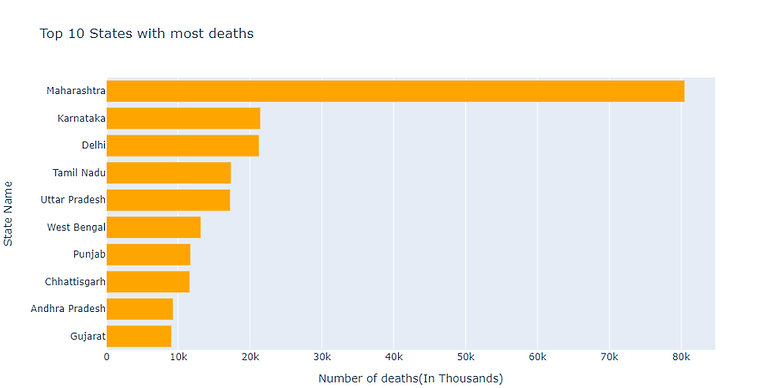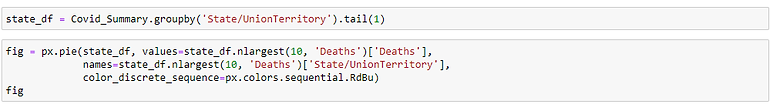Fig: Top 10 states with highest death cases (in percentage)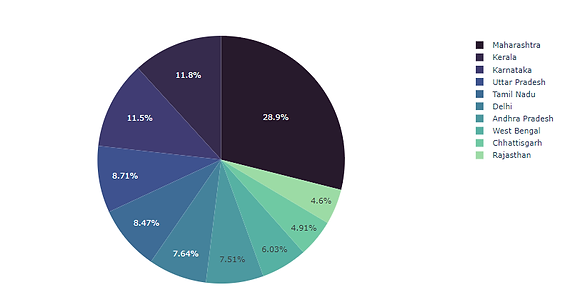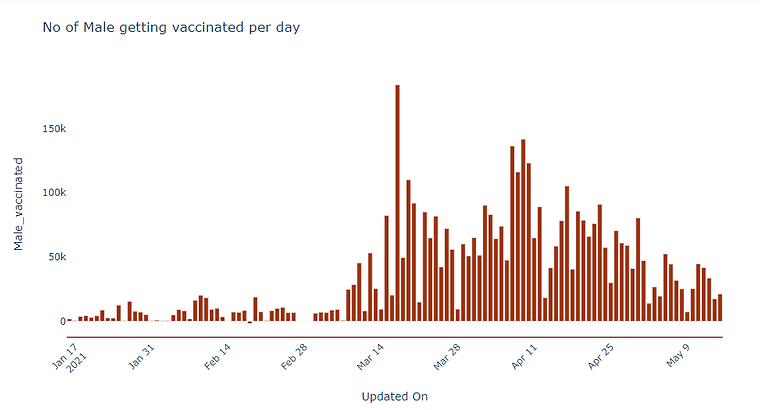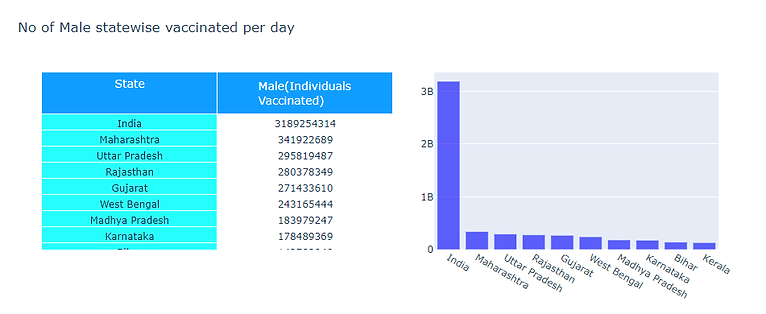Usually, in the basic ARIMA model, we need to provide the p, d, and q values which are essential. We use statistical techniques to generate these values by performing the difference to eliminate the non-stationarity and plotting ACF and PACF graphs. In Auto ARIMA, the model itself will generate the optimal p, d, and q values which would

be suitable for the data set to provide better forecasting.

Once fitting the whole dataset into auto_arima model, it will generate the best fit values of p, d and q which is suitable for our dataFinalized the best model – ARIMA (5, 1, 2) – (p, d, q)

Last 3 zeros (0, 0, 0) represents that, our data does not have any seasonality.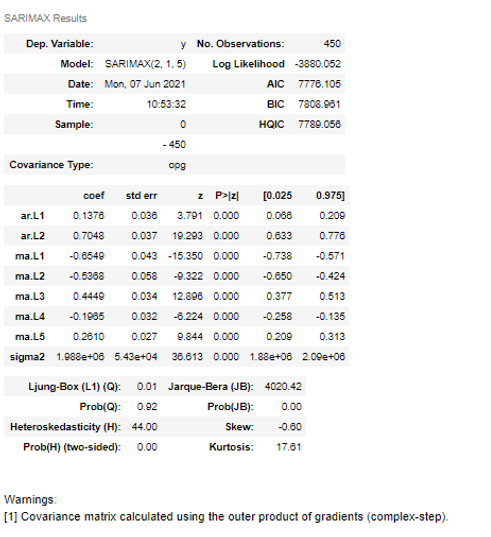Fig: Summary of the modelNow, fitting the ARIMA model with whole data with best fit value of p, d and q to check how accurately our model is getting trained.Fig: Graphical representation Actual vs PredictionFig: comparing actual cases vs prediction

# Checking Accuracy and Error

Mean Absolute Percentage Error (MAPE)

# The MAPE has an advantage over MAE or RMSE as it is unit-free and thus is safe to use for comparing performances of time series forecast values with different units.# Prediction# Again, fitting the data into the arima model and get trained# Predicting the confirmed cases for next two weeks from the current date using the above trained modelUsing the following above steps, we able to train and test our model for confirmed cases, death cases, cured cases, gender wise getting vaccination, brand wise vaccination (covaxin & covishield)

Let’s reorganize this set of predictions by creating a dataframe that contains our future forecastNow that we’ve evaluated our data and satisfied with the performance, the next step would be to refit our model to all the states and then forecast into the real future.

bottom of page